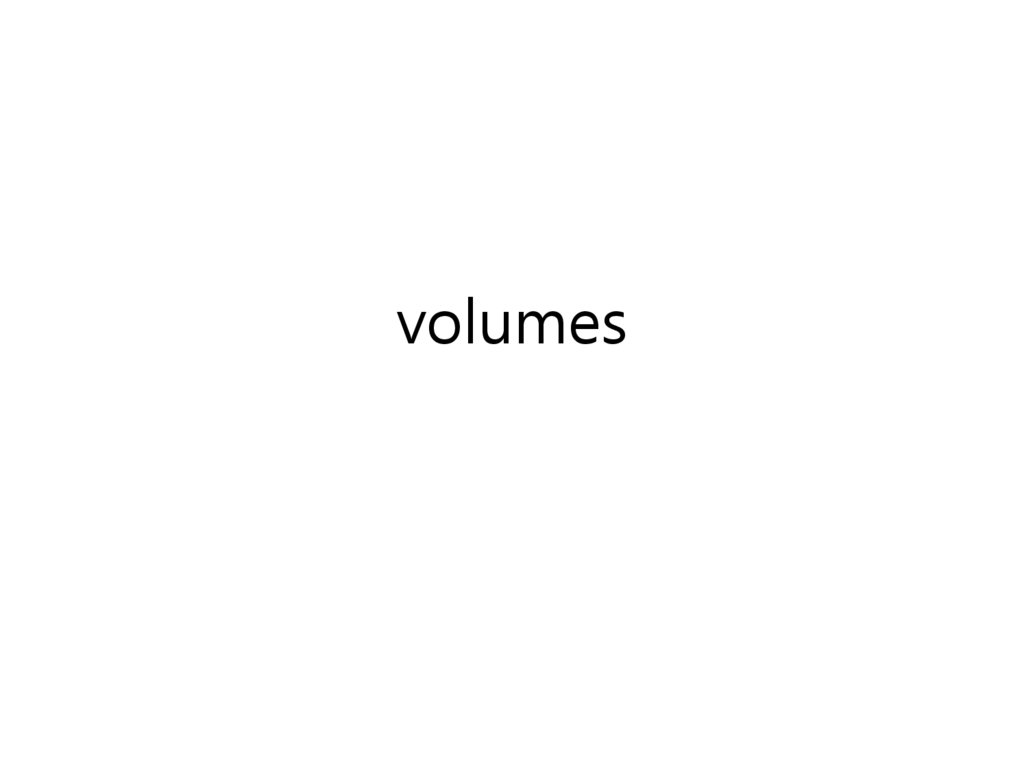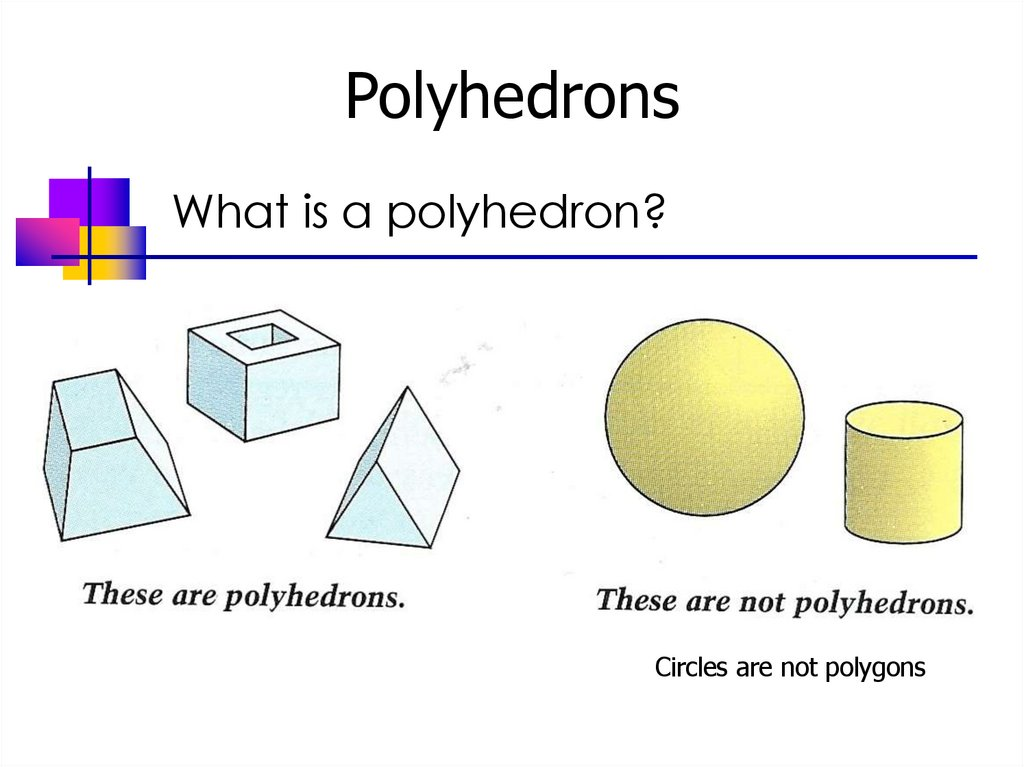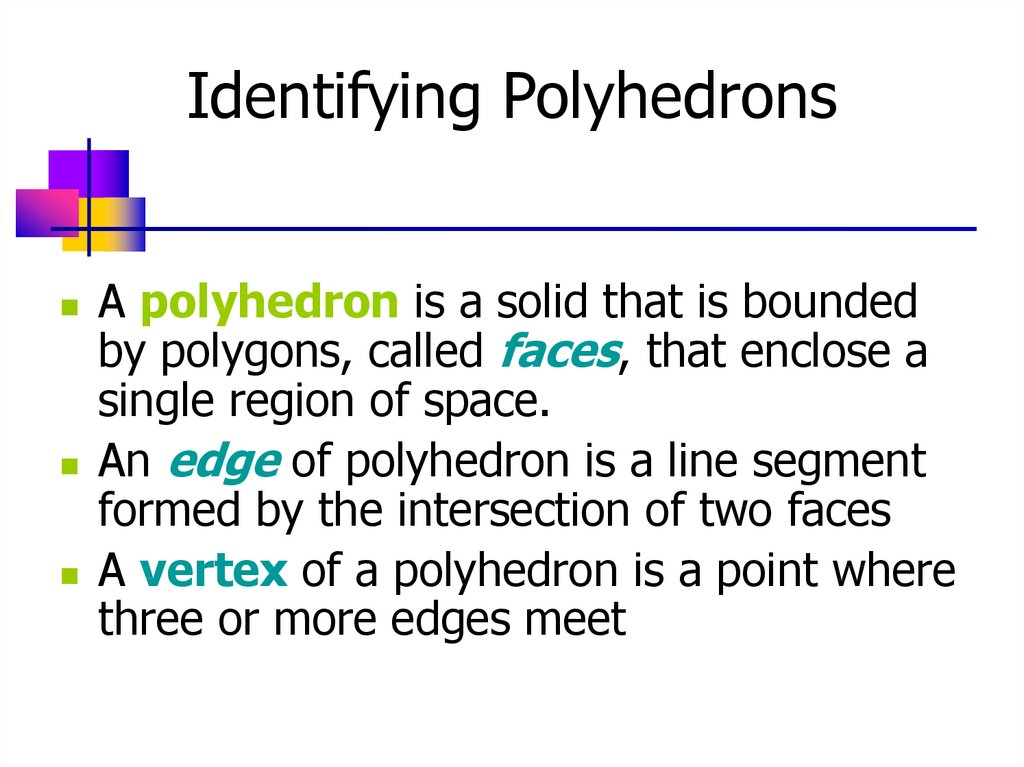Surface area and volumes

2. Polyhedrons

What is a polyhedron?
Circles are not polygons

3. Identifying Polyhedrons

A polyhedron is a solid that is bounded
by polygons, called faces, that enclose a
single region of space.
An edge of polyhedron is a line segment
formed by the intersection of two faces
A vertex of a polyhedron is a point where
three or more edges meet

5. Example 1 Counting Faces, Vertices, and Edges

Count the faces, vertices, and
edges of each polyhedron

6.

Example 1A
Counting Faces
Count the faces, vertices, and edges of each polyhedron
4 faces

7.

Example 1a
Counting Vertices
Count the faces, vertices, and edges of each polyhedron
4 vertices

8.

Example 1a
Counting Edges
Count the faces, vertices, and edges of each polyhedron
6 edges

9.

Example 1b
Counting Faces
Count the faces, vertices, and edges of each polyhedron
5 faces

10.

Example 1b
Counting Vertices
Count the faces, vertices, and edges of each polyhedron
5 vertices

11.

Example 1b
Counting Vertices
Count the faces, vertices, and edges of each polyhedron
8 edges

12.

Example 1c
Counting Faces
Count the faces, vertices, and edges of each polyhedron
6 faces

13.

Example 1c
Counting Vertices
Count the faces, vertices, and edges of each polyhedron
6 vertices

14.

Example 1c
Counting Edges
Count the faces, vertices, and edges of each polyhedron
10 edges

15.

Notice a Pattern?
Faces
Vertices
Edges
4
4
6
5
5
8
6
6
10

16.

Theorem 12.1
Euler's Theorem
The number of faces (F), vertices (V),
and edges (E) of a polyhedron is related
by F + V = E + 2

17.

The surface of a polyhedron consists of all
points on its faces
A polyhedron is convex if any two points on
its surface can be connected by a line
segment that lies entirely inside or on the
polyhedron

18.

Regular Polyhedrons
A polyhedron is regular if all its faces are
congruent regular polygons.
Not regular
3 faces
4 faces
regular
Vertices are
not formed
by the same
number of
faces

19.

5 kinds of Regular
Polyhedrons
6 faces
4 faces
12 faces
8 faces
20 faces

20.

Example 2
Classifying Polyhedrons
One of the octahedrons is regular.
Which is it?
A polyhedron is regular if all its faces are congruent regular polygons.

21.

Example 2
Classifying Polyhedrons
All its faces are
congruent equilateral
triangles, and each
vertex is formed by
the intersection of 4
faces
Faces are not
all congruent
(regular
hexagons and
squares)
Faces are not all
regular polygons
or congruent
(trapezoids and
triangles)

22.

Example 3
Counting the Vertices of a Soccer Ball
A soccer ball has 32 faces: 20 are
regular hexagons and 12 are regular
pentagons. How many vertices does it
have?
A soccer ball is an example of a
semiregular polyhedron - one
whose faces are more than one
type of regular polygon and whose
vertices are all exactly the same

23.

Example 3
Counting the Vertices of a Soccer Ball
A soccer ball has 32 faces: 20 are regular hexagons and 12 are
regular pentagons. How many vertices does it have?
Hexagon = 6 sides, Pentagon = 5 sides
Each edge of the soccer ball is shared by two sides
Total number of edges = ½(6●20 + 5●12)= ½(180)= 90
Now use Euler's Theorem
F+V=E+2
32 + V = 90 + 2
V = 60

24.

Prisms
A prism is a polyhedron that
has two parallel, congruent
faces called bases.
The other faces, called lateral
faces, are parallelograms and
are formed by connecting
corresponding vertices of the
bases
The segment connecting these
corresponding vertices are
lateral edges
Prisms are classified by their
bases
base

25.

Prisms
The altitude or height, of a
prism is the perpendicular
distance between its bases
In a right prism, each
lateral edge is
perpendicular to both
bases
Prisms that have lateral
edges that are oblique
(≠90°) to the bases are
oblique prisms
The length of the oblique
lateral edges is the slant
height of the prism

26.

Surface Area of a Prism
The surface area of a
polyhedron is the sum of the
areas of its faces

27.

Example 1
Find the Surface Area of a Prism
The
Skyscraper
is 414 meters
high. The base is
a square with
sides that are 64
meters. What is
the surface area
of the
skyscraper?

28.

Example 1
Find the Surface Area of a Prism
The Skyscraper is 414 meters high. The base is a square with sides that are
64 meters. What is the surface area of the skyscraper?
64(64)=4096
64(64)=4096
64(414)=26496
414
64(414)=26496
64(414)=26496
64(414)=26496
64
64
Surface Area = 4(64•414)+2(64•64)=114,176 m2

29.

Example 1
Find the Surface Area of a Prism
The Skyscraper is 414 meters high. The base is a square with sides that are
64 meters. What is the surface area of the skyscraper?
Surface Area = 4(64•414)+2(64•64)=114,176 m2
Surface Area = (4•64)•414+2(64•64)=114,176 m2
height
Perimeter of
the base
414
Area of
the base
64
64

30.

Nets
A net is a pattern that can be cut
and folded to form a polyhedron.
A
B
C
D
F
A
D
E
E

31.

Surface Area of a Right Prism
The surface area, S, of a right prism is
S = 2B + Ph
where B is the area of a base, P is the
perimeter of a base, and h is the height

32.

Example 2
Finding the Surface Area of a Prism
Find the surface area of each right prism
12 in.
8 in.
4 in.
5 in.
12 in.
5 in.

33.

Example 2
Finding the Surface Area of a Prism
Find the surface area of each right prism
S = 2B + Ph
Area of the Base = 5x12=60
8 in.
Perimeter of Base = 5+12+5+12 = 34
5 in.
12 in.
Height of Prism = 8
•S = 2B + Ph
•S = 2(60) +(34)•8
•S = 120 + 272 = 392 in2

34.

Example 2
Finding the Surface Area of a Prism
Find the surface area of each right prism
S = 2B + Ph
12 in.
Area of the Base = ½(5)(12)=30
4 in.
Perimeter of Base = 5+12+13=30
5 in.
Height of Prism = 4
(distance between triangles)
•S = 2B + Ph
•S = 2(30) +(30)•4
•S = 60 + 120 = 180 in2

35.

Cylinders
A cylinder is a solid with
congruent circular bases that lie
in parallel planes
The altitude, or height, of a
cylinder is the perpendicular
distance between its bases
The lateral area of a cylinder
is the area of its curved lateral
surface.
A cylinder is right if the
segment joining the centers of
its bases is perpendicular to its
bases

36.

Surface Area of a Right Cylinder
The surface area, S, of a right circular
cylinder is
S = 2B + Ch
or
2πr2 + 2πrh
where B is the area of a base, C is the circumference
of a base, r is the radius of a base, and h is the height

37.

Example 3
Finding the Surface Area of a Cylinder
Find the surface area of the cylinder
3 ft
4 ft

38.

Example 3
Finding the Surface Area of a Cylinder
Find the surface area of the cylinder
2πr2 + 2πrh
2π(3)2 + 2π(3)(4)
18π + 24π
42π ≈ 131.9 ft2
Height = 4
3 ft
4 ft

39.

Pyramids
A pyramid is a polyhedron in which
the base is a polygon and the
lateral faces are triangles that
have a common vertex

40.

Pyramids
The intersection of
two lateral faces is a
lateral edge
The intersection of the
base and a lateral
face is a base edge
The altitude or height
of the pyramid is the
perpendicular
distance between the
base and the vertex

41.

Regular Pyramid
A pyramid is regular if its base is a regular
polygon and if the segment from the
vertex to the center of the base is
perpendicular to the base
The slant height of a regular pyramid is
the altitude of any lateral face (a nonregular
pyramid has no slant height)

42.

Developing the formula for surface
area of a regular pyramid
Area of each triangle is ½bL
Perimeter of the base is 6b
Surface Area =
(Area of base) + 6(Area of lateral faces)
S = B + 6(½bl)
S = B + ½(6b)(l)
S= B + ½Pl

43.

Surface Area of a Regular Pyramid
The surface area, S, of a regular
pyramid is
S = B + ½Pl
Where B is the area of the base, P is
the perimeter of the base, and L is
the slant height

44.

Example 1
Finding the Surface Area of a Pyramid
Find the surface area of each
regular pyramid

45.

Example 1
Finding the Surface Area of a Pyramid
Find the surface area of each regular
pyramid
S = B + ½PL
Base is a Square
Area of Base = 5(5) = 25
Perimeter of Base
5+5+5+5 = 20
Slant Height = 4
S = 25 + ½(20)(4)
= 25 + 40
= 65 ft2

46.

Example 1
Finding the Surface Area of a Pyramid
Find the surface area of each regular pyramid
S = B + ½PL
Base is a Hexagon
A=½aP
1
A (3 3)(36) 54 3
2
Perimeter = 6(6)=36
S = 54 3 + ½(36)(8)
= 54 3 + 144
= 237.5 m2
Slant Height = 8

47.

Cones
A cone is a solid that has a
circular base and a vertex that is
not in the same plane as the base
The lateral surface consists of all
segments that connect the vertex
with point on the edge of the
base
The altitude, or height, of a cone
is the perpendicular distance
between the vertex and the
plane that contains the base

48.

Right Cone
A right cone is one in which the
vertex lies directly above the center
of the base
The slant height of a right cone is
the distance between the vertex
and a point on the edge of the
base

49.

Developing the formula for the surface
area of a right cone
Use the formula for surface
area of a pyramid S = B +
½Pl
As the number of sides on
the base increase it
becomes nearly circular
Replace ½P (half the
perimeter of the pyramids
base) with πr (half the
circumference of the cone's base)

50.

Surface Area of a Right Cone
The surface area, S, of a right cone is
S = πr2 + πrl
Where r is the radius of the base and L is
the slant height of the cone

51. Example 2 Finding the Surface Area of a Right Cone

Find the surface area of the right
cone

52. Example 2 Finding the Surface Area of a Right Cone

• Find the surface area of the right
cone
S = πr2 + πrl
= π(5)2 + π(5)(7)
= 25π + 35π
= 60π or 188.5 in2
Slant height = 7

53. Volume formulas

The Volume, V, of a prism is V = Bh
The Volume, V, of a cylinder is V = πr2h
The Volume, V, of a pyramid is V = 1/3Bh
The Volume, V, of a cone is V = 1/3πr2h
The Surface Area, S, of a sphere is S = 4πr2
The Volume, V, of a sphere is V = 4/3πr3

54.

Volume
• The volume of a polyhedron is the
number of cubic units contained in its
interior
• Label volumes in cubic units like cm3,
in3, ft3, etc

55.

Postulates
• All the formulas for the volumes of
polyhedrons are based on the
following three postulates
– Volume of Cube Postulate: The volume of
a cube is the cube of the length of its
side, or V = s3
– Volume Congruence Postulate: If two
polyhedrons are congruent, then they
have the same volume
– Volume Addition Postulate: The volume
of a solid is the sum of the volumes of
all its nonoverlapping parts

56.

Example 1: Finding the Volume of
a Rectangular Prism
• The cardboard box is 5" x 3" x 4"
How many unit cubes can be packed
into the box? What is the volume of
the box?

57.

Example 1: Finding the Volume of a
rectangular Prism
• The cardboard box is 5" x 3" x 4" How many unit cubes can
be packed into the box? What is the volume of the box?
•How many cubes in bottom
layer?
•5(3) = 15
•How many layers?
•4
•V=5(3)(4) = 60 in3
V = L x W x H for a rectangular prism

58.

Volume of a Prism and a
Cylinder
Cavalieri's Principle
If two solids have the
same height and the same
cross-sectional area at
every level, then they have
the same volume

59.

Volume of a Prism
• The Volume, V, of a prism is
V = Bh
where B is the area of a base and h is
the height

60.

Volume of a Cylinder
• The volume, V, of a cylinder is V=Bh or
V = πr2h
where B is the area of a base, h is the height
and r is the radius of a base

61.

Example 2
Finding Volumes
• Find the volume of the right prism
and the right cylinder

62.

Example 2
Finding Volumes
• Find the volume of the right prism
and the right cylinder
Area of Base
B = ½(3)(4)=6
Height = 2
V = Bh
V = 6(2)
V = 12 cm3
3 cm
4 cm

63.

Example 2
Finding Volumes
• Find the volume of the right prism
and the right cylinder
Area of Base
B = π(7)2 =49π
Height = 5
V = Bh
V = 49π(5)
V = 245π in3

64.

Example 3
Estimating the Cost of Moving
• You are moving from Newark, New Jersey, to Golden,
other belongings will fill half the truck trailer. The
moving company estimates that your belongings weigh
an average of 6.5 pounds per cubic foot. The company
charges \$600 to ship 1000 pounds. Estimate the cost

65.

Example 3
Estimating the Cost of Moving
You are moving from Newark, New Jersey, to Golden, Colorado - a trip of
2000 miles. Your furniture and other belongings will fill half the truck
trailer. The moving company estimates that your belongings weigh an
average of 6.5 pounds per cubic foot. The company charges \$600 to ship
1000 pounds. Estimate the cost of shipping your belongings.
Volume = L x W x H
V = 50(8)(9)
V= 3600 ft3
3600 ÷ 2 = 1800 ft3
1800(6.5) = 11,700 pounds
11,700 ÷ 1000 = 11.7
11.7(600) = \$7020